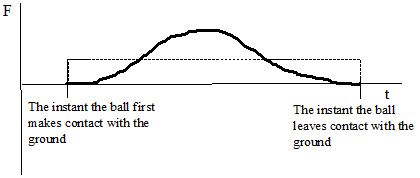# 02. Model Specifics

$$\newcommand{\vecs}{\overset { \rightharpoonup} {\mathbf{#1}} }$$ $$\newcommand{\vecd}{\overset{-\!-\!\rightharpoonup}{\vphantom{a}\smash {#1}}}$$$$\newcommand{\id}{\mathrm{id}}$$ $$\newcommand{\Span}{\mathrm{span}}$$ $$\newcommand{\kernel}{\mathrm{null}\,}$$ $$\newcommand{\range}{\mathrm{range}\,}$$ $$\newcommand{\RealPart}{\mathrm{Re}}$$ $$\newcommand{\ImaginaryPart}{\mathrm{Im}}$$ $$\newcommand{\Argument}{\mathrm{Arg}}$$ $$\newcommand{\norm}{\| #1 \|}$$ $$\newcommand{\inner}{\langle #1, #2 \rangle}$$ $$\newcommand{\Span}{\mathrm{span}}$$ $$\newcommand{\id}{\mathrm{id}}$$ $$\newcommand{\Span}{\mathrm{span}}$$ $$\newcommand{\kernel}{\mathrm{null}\,}$$ $$\newcommand{\range}{\mathrm{range}\,}$$ $$\newcommand{\RealPart}{\mathrm{Re}}$$ $$\newcommand{\ImaginaryPart}{\mathrm{Im}}$$ $$\newcommand{\Argument}{\mathrm{Arg}}$$ $$\newcommand{\norm}{\| #1 \|}$$ $$\newcommand{\inner}{\langle #1, #2 \rangle}$$ $$\newcommand{\Span}{\mathrm{span}}$$$$\newcommand{\AA}{\unicode[.8,0]{x212B}}$$

# Model Specifics

For our first pass through the study of mechanics, we will make a set of simplifying assumptions leading to a model of reality termed the one-dimensional, constant-force, particle model. We will assume that:

## The object moves along a perfectly straight line

Thus, if we are studying the motion of a car we will assume that the road is perfectly smooth with no bumps, the road is perfectly straight with no curves, and the driver is perfectly steady at the wheel with no swaying to the right or left.

## The object is acted on by constant forces

For example, when a basketball bounces off the ground the force of the ground on the basketball is:

• zero before the basketball strikes the ground,
• non-zero and increasing in magnitude as the basketball deforms in its contact with the ground,
• non-zero and decreasing in magnitude as the basketball springs back into shape while still in contact with the ground,
• and once again zero as the ball leaves contact with the ground.

In reality, the force between the basketball and the ground is quite complicated.

However, in our model we will approximate the force on the basketball due to the ground as:

• zero before the basketball strikes the ground,
• non-zero and constant in magnitude the entire time the ball is in contact with the ground,
• and once again zero as the ball leaves contact with the ground.

The difference between reality and our model is illustrated by the graph below. The dark line represents the actual force between the ground and the ball, the dashed line represents how we will approximate the force in our current model.In addition, you will soon learn that the total force acting on an object is proportional to its acceleration. Thus, in this model, we will approximate all accelerations as constant.

## The object’s size and shape are unimportant

In physics, the word particle refers to a hypothetical entity having no size and therefore no shape. The statement that objects will be thought of as particles simply means that we will ignore any effects their actual size and shape could have on the scenarios we investigate.

## The object is classical

For this entire course, we will assume the objects under investigation are much larger than an atom and move much slower than the speed of light.

This page titled 02. Model Specifics is shared under a CC BY-NC-SA license and was authored, remixed, and/or curated by Paul D'Alessandris.